CBSE Class 12 Sample Paper for 2022 Boards (MCQ Based - for Term 1)

Class 12
Solutions of Sample Papers and Past Year Papers - for Class 12 Boards

## (a) ±  1  (b) −1      (c) ±  1/2  (d) 1/2

This question is inspired from -   Question 21 - CBSE Class 12 Sample Paper for 2021 Boards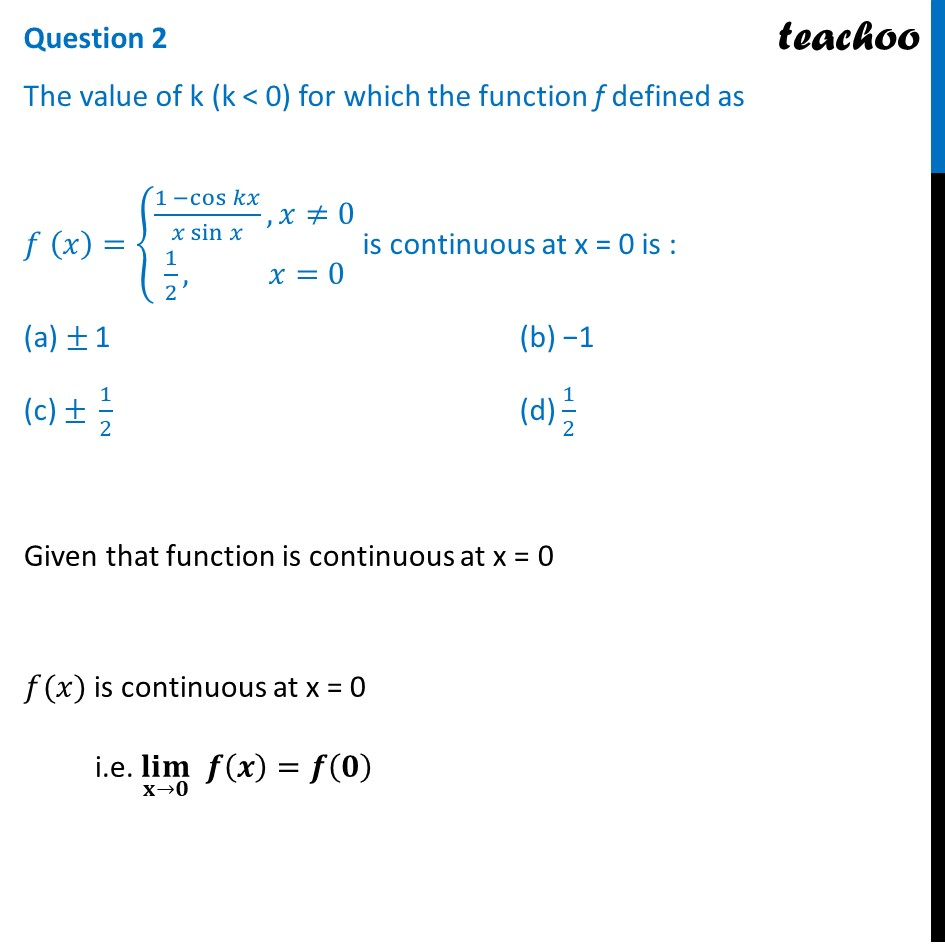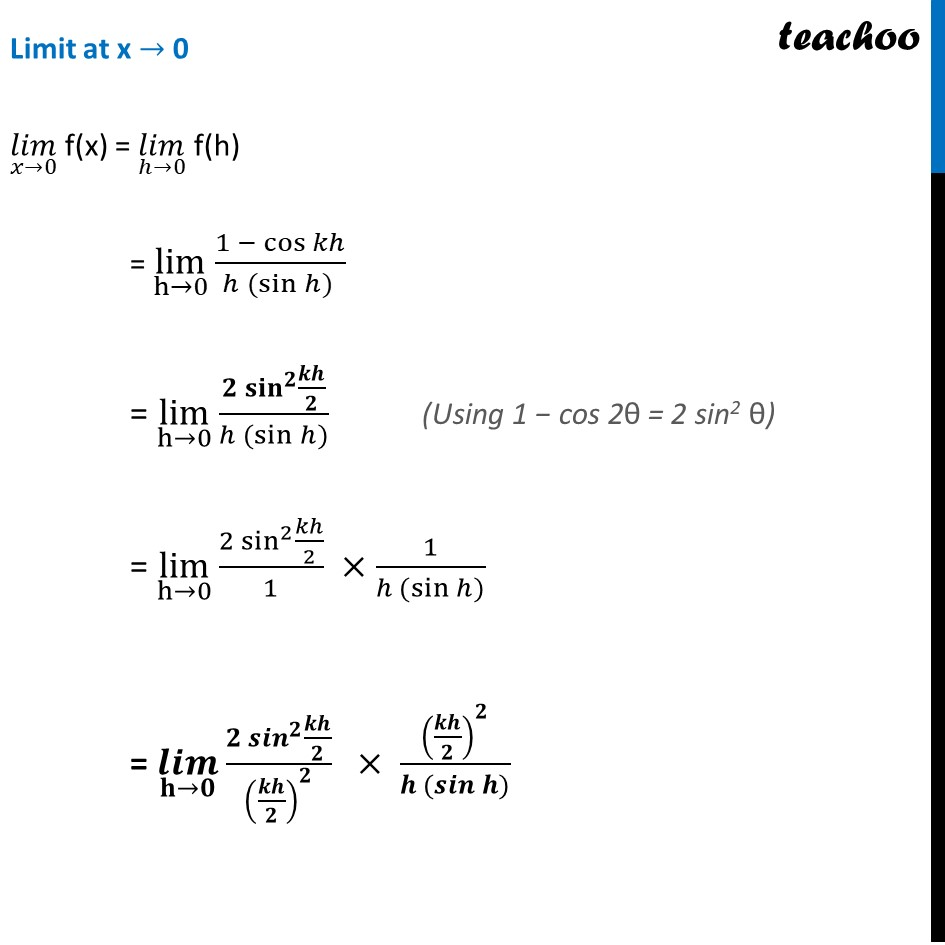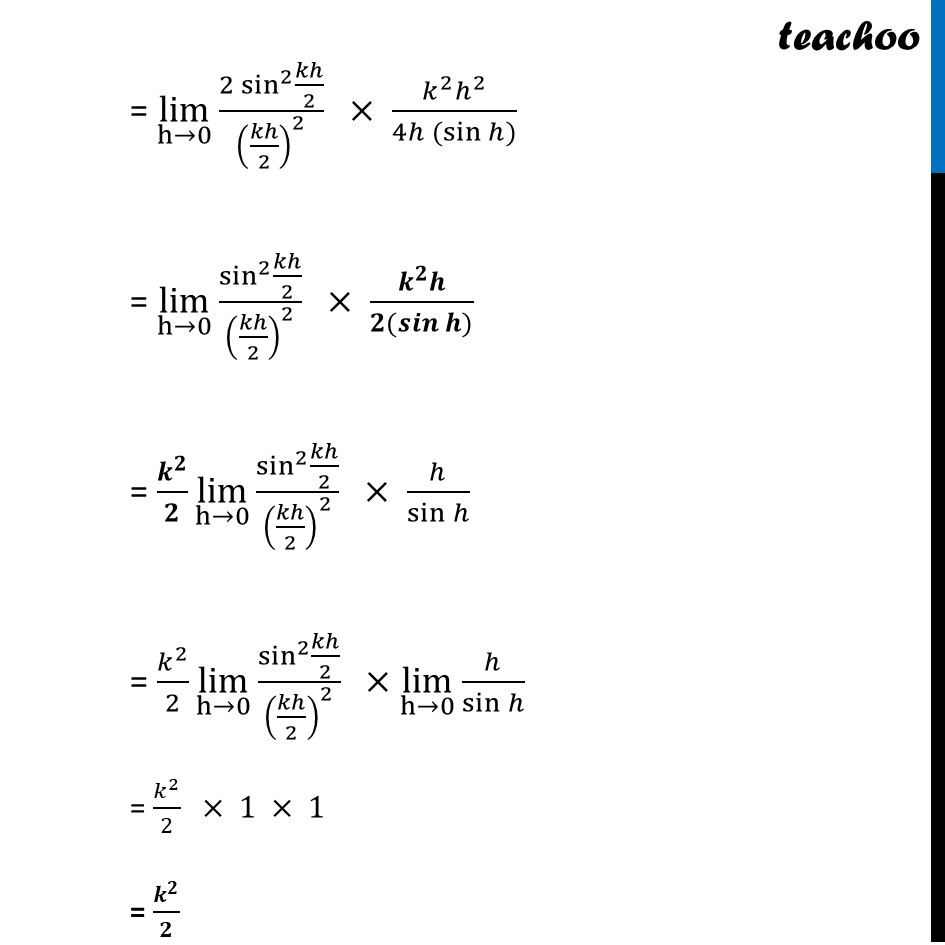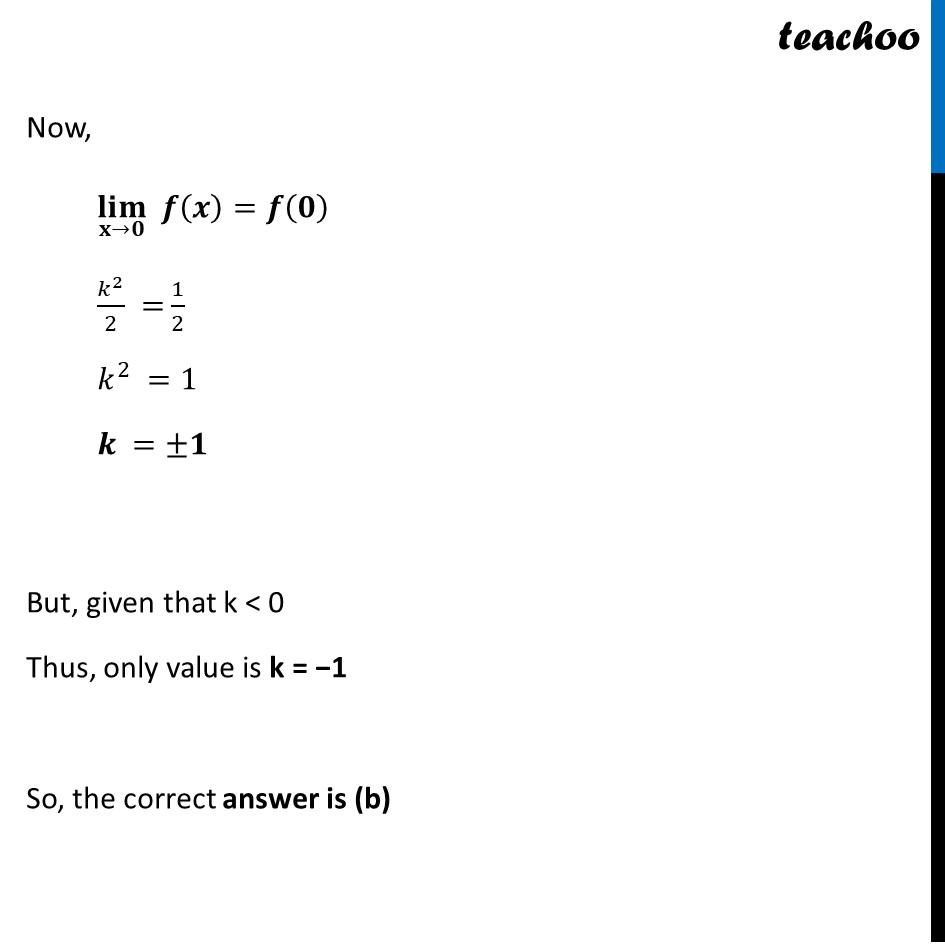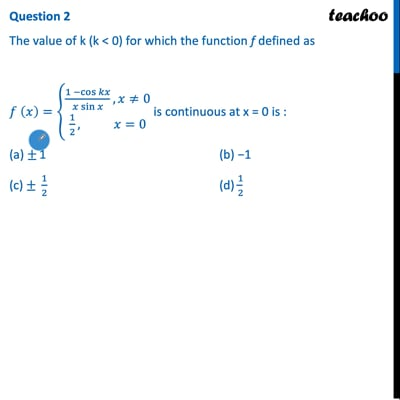This video is only available for Teachoo black users

Introducing your new favourite teacher - Teachoo Black, at only ₹83 per month

### Transcript

Question 2 The value of k (k < 0) for which the function f defined as 𝑓 (𝑥)={█((1 −cos⁡𝑘𝑥)/(𝑥 sin⁡𝑥 ),𝑥≠0@1/2, 𝑥=0)┤ is continuous at x = 0 is : (a) ± 1 (b) −1 (c) ± 1/2 (d) 1/2 Given that function is continuous at x = 0 𝑓(𝑥) is continuous at x = 0 i.e. (𝐥𝐢𝐦)┬(𝐱→𝟎) 𝒇(𝒙)=𝒇(𝟎) Limit at x → 0 (𝑙𝑖𝑚)┬(𝑥→0) f(x) = (𝑙𝑖𝑚)┬(ℎ→0) f(h) = lim┬(h→0) (1 − cos⁡𝑘ℎ)/(ℎ (sin⁡ℎ) ) = lim┬(h→0) (𝟐 〖𝐬𝐢𝐧〗^𝟐⁡〖𝒌𝒉/𝟐〗)/(ℎ (sin⁡ℎ)) = lim┬(h→0) (2 sin^2⁡〖𝑘ℎ/2〗)/1 ×1/(ℎ (sin⁡ℎ)) = (𝒍𝒊𝒎)┬(𝐡→𝟎) (𝟐 〖𝒔𝒊𝒏〗^𝟐⁡〖𝒌𝒉/𝟐〗)/(𝒌𝒉/𝟐)^𝟐 × (𝒌𝒉/𝟐)^𝟐/(𝒉 (𝒔𝒊𝒏⁡𝒉)) = lim┬(h→0) (2 sin^2⁡〖𝑘ℎ/2〗)/(𝑘ℎ/2)^2 × (𝑘^2 ℎ^2)/(4ℎ (sin⁡ℎ)) = lim┬(h→0) sin^2⁡〖𝑘ℎ/2〗/(𝑘ℎ/2)^2 × (𝒌^𝟐 𝒉)/(𝟐(𝒔𝒊𝒏⁡𝒉)) = 𝒌^𝟐/𝟐 lim┬(h→0) sin^2⁡〖𝑘ℎ/2〗/(𝑘ℎ/2)^2 × ℎ/sin⁡ℎ = 𝑘^2/2 lim┬(h→0) sin^2⁡〖𝑘ℎ/2〗/(𝑘ℎ/2)^2 ×lim┬(h→0) ℎ/sin⁡ℎ = 𝑘^2/2 × 1 × 1 = 𝒌^𝟐/𝟐 Now, (𝐥𝐢𝐦)┬(𝐱→𝟎) 𝒇(𝒙)=𝒇(𝟎) 𝑘^2/2 = 1/2 𝑘^2 =1 𝒌 =±𝟏 But, given that k < 0 Thus, only value is k = −1 So, the correct answer is (b)